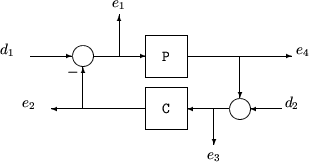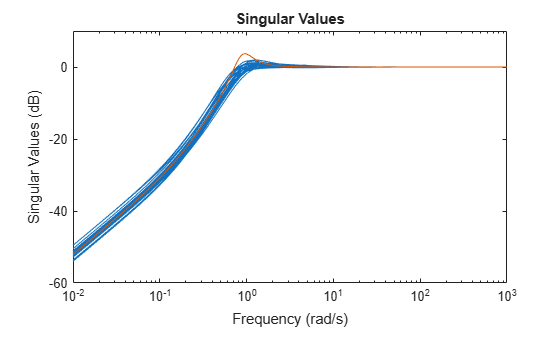## Worst-Case Sensitivity Functions of Feedback Loops

The sensitivity function and the complementary sensitivity function are two transfer functions related to the robustness and performance of a closed-loop system. Consider a general multivariable closed-loop control structure, as in the following illustration.The following table gives the values of the input and output sensitivity functions for this control structure.

Description

Equation

Input sensitivity Si (closed-loop transfer function from d1 to e1)

Si = (I + CP)–1

Input complementary sensitivity Ti (closed-loop transfer function from d1 to e2)

Ti = CP(I + CP)–1

Output sensitivity So (closed-loop transfer function from d2 to e3)

So = (I + PC)–1

Output complementary sensitivity To (closed-loop transfer function from d2 to e4)

To = PC(I + PC)–1

Input loop transfer function Li

Li = CP

Output loop transfer function Lo

Lo = PC

### Worst-Case Sensitivity and Complementary Sensitivity

When you have an uncertain plant model and a controller model, you can compute the worst-case sensitivity and complementary sensitivity functions for robustness analysis. To do so, construct the transfer function you want to evaluate and use `wcgain` to find the perturbations that yield the worst-case gain for that transfer function. Then, use `usubs` to compute the transfer function corresponding to that worst-case gain.

For this example, create a SISO uncertain plant P and a PID controller.

```delta = ultidyn('delta',[1 1]); tau = ureal('tau',5,'range',[4 6]); P = tf(1,[tau 1])*(1+0.25*delta); C = pid(4,4);```

Construct the uncertain sensitivity and complementary sensitivity transfer functions, ${{\mathit{S}}_{\mathit{i}}=\left(\mathit{I}+\mathrm{CP}\right)}^{-1}$ and ${\mathit{T}}_{\mathit{i}}=\mathit{I}-{\mathit{S}}_{\mathit{i}}$, respectively. (For this SISO system, the input and output sensitivity functions are equal.)

```Si = feedback(1,C*P); Ti = 1 - Si;```

Compute the worst-case peak gains of `Si` and `Ti` and the corresponding worst-case perturbations using `wcgain`.

```[wcgS,wcuS] = wcgain(Si); [wcgT,wcuT] = wcgain(Ti);```

Finally, evaluate the sensitivity functions with these worst-case perturbations.

```Siwc = usubs(Si,wcuS); Tiwc = usubs(Ti,wcuT);```

`Siwc` and `Tiwc` are the worst-case sensitivity and complementary sensitivity functions for the uncertainty specified in the plant. Examine the effect of uncertainty on the sensitivity function `Si` by plotting the frequency response of some samples. The actual worst-case peak gain `wcgS` can be significantly higher than the random samples show.

```rng(0); % for reproducibility sigma(Si,Siwc)```